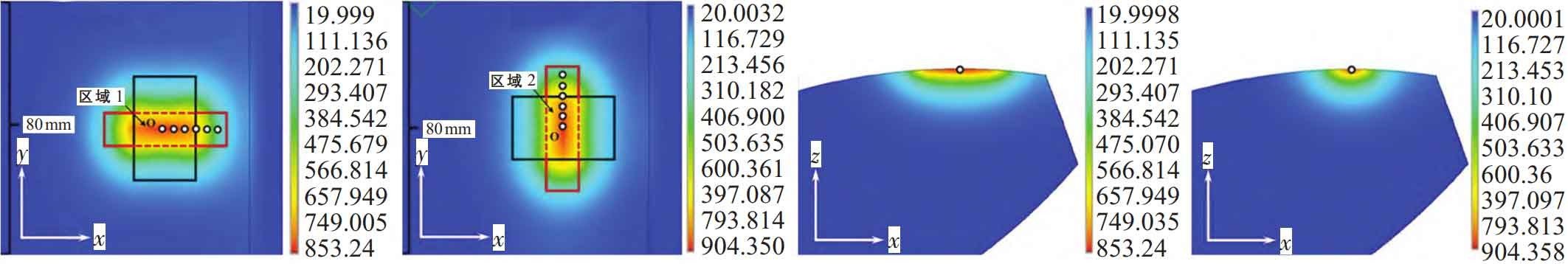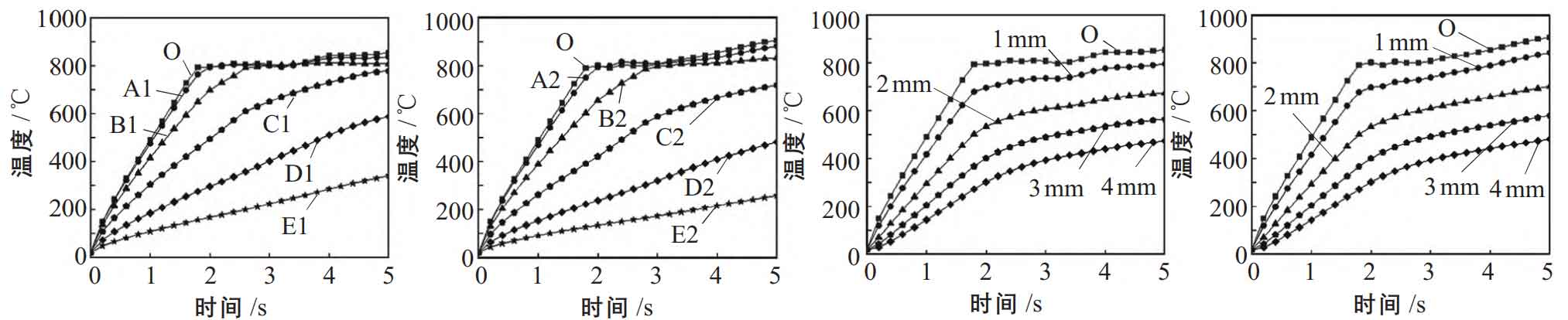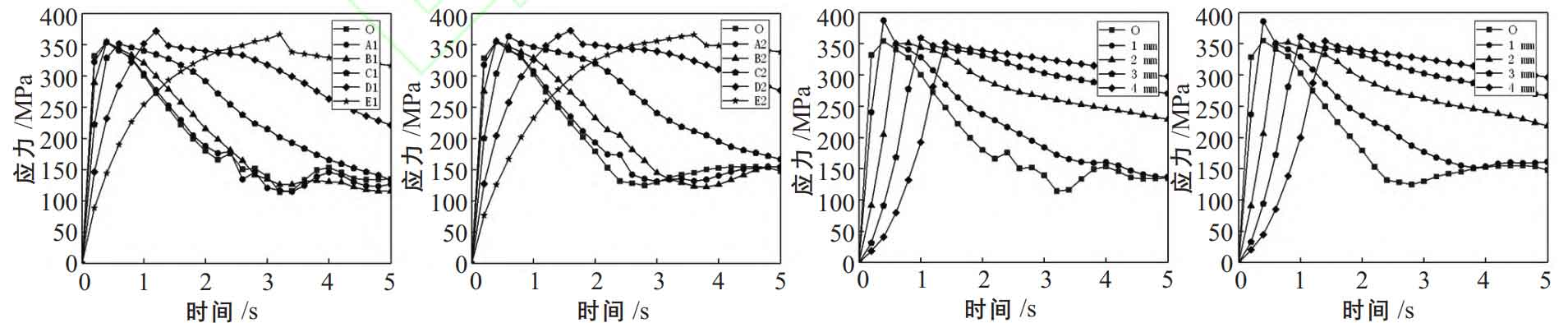# Simulation results and discussion of induction heating of large spur gears

Figure 1 shows the front view of the temperature field on the single tooth profile surface and the middle section temperature field in the end face direction after heating for 5 s in two schemes. It can be seen that the heat affected zone of the two schemes is located directly below the inductor, and the maximum temperature appears in the center of the heat affected zone. In order to facilitate the analysis, multiple observation points can be selected for analysis as shown in Figure 1. In Figure 1 (a), point O is the center point projected by the sensor on the tooth profile, and the points in the X direction of point o are A1, B1, C1, D1 and E1 in turn, with the same distance. In Fig. 1 (b), point O is also the center point of the projection of the sensor on the tooth profile, and the points in the Y direction of point o are A2, B2, C2, D2 and E2, with equal distances. In Figure 1 (c) and (d), four points in the negative direction of o point Z are selected as observation points, with a distance of 1mm, which are not marked in the figure.(a) Scheme 1 tooth profile (b) scheme 2 tooth profile (c) section in scheme 1 (d) section in scheme 2

Figure 2 shows the temperature change trend at different observation points. It can be seen that the temperature change trend of each observation point of the two schemes is the same. At the initial stage of heating, the temperature of each observation point rises rapidly, and the heating rate of point O is the fastest. At 1.7 s, the temperature reaches 768 ℃, reaching the Curie temperature. The material loses magnetism and the eddy current density decreases sharply, but there is still eddy current. Therefore, the temperature rise rate slows down sharply but still rises. The temperature rise rate of other observation points decreases with the increase of the distance from point O, and the temperature rise rate of the points (points c, D and E) outside the coil covered by the guide magnet decreases significantly, because the eddy current intensity of the coil covered by the guide magnet is high on the surface of the tooth profile, and the eddy current intensity of the coil outside the coverage is weak on the surface of the tooth profile. In the Z direction, at the beginning of heating, because the induced eddy current is mainly distributed on the tooth profile surface, the temperature rise rate of point O is the largest, and the temperature rise rate of other observation points decreases with the increase of the distance from point o.(a) Plan 1 surface points (b) plan 2 surface points (c) plan 1 Z direction points (d) plan 2 Z direction points

Fig. 3 shows the changes of temperature standard deviation and uniformity during the heating process of the two schemes. The observation areas selected in scheme 1 and scheme 2 are region 1 and region 2 in Figure 1 respectively. As can be seen from Fig. 3, the temperature uniformity of the gear before heating in the two schemes is 100%. At the initial stage of heating, due to the inconsistent heating rate at each point, the temperature difference is large, and the temperature uniformity decreases sharply. As the heat conduction and eddy current tend to be stable, the increase rate of temperature difference at each point slows down, and the decrease rate of temperature uniformity slows down. After heating to 1.7 s, because the temperature in some areas reaches the Curie temperature, the temperature change is very small, the temperature in other areas continues to rise, the temperature difference decreases, the temperature uniformity begins to rise, and basically tends to be stable after 2.7 s. In the whole heating process, the temperature uniformity of scheme 1 is greater than that of scheme 2. At the 5th s, the temperature uniformity is 92.62% and 90.28% respectively.

Fig. 4 shows the stress variation trend of gears at different observation points during induction heating. The overall change trend of stress at each observation point is first increased and then decreased. The thermal stress is caused by the non-uniformity of temperature, and the yield limit of the material also affects its variation trend. For example, at point O, the temperature uniformity of the tooth profile surface is poor at the initial stage of heating, and the thermal stress rises rapidly. The stress reaches the maximum at 0.4 s, which is 354.16 MPa (scheme 1) and 355.11 MPa (scheme 2) respectively, reaching the yield limit of the material. As the temperature continues to rise, the yield limit of the material further decreases and the thermal stress decreases, because the thermal stress exceeds the yield limit of the material and produces plastic deformation. After that, the change trend of temperature and temperature uniformity decreases, and the thermal stress is basically unchanged. The rate of increase and decrease of thermal stress at other observation points decreases with the increase of distance from point O, and the maximum value of thermal stress is large because the temperature of D1 and E1 points is small and the yield limit is large. In the Z direction, the change of thermal stress at each observation point also increases first and then decreases. The rise rate of thermal stress decreases with the increase of distance from o point, and the decline rate increases with the increase of distance from o point.(a) Plan 1 surface points (b) plan 2 surface points (c) plan 1 Z direction points (d) plan 2 Z direction points

Figure 5 shows the thermal stress standard deviation and its uniformity of the two schemes. As can be seen from Fig. 5, the uniformity of temperature and thermal stress of gear during induction heating of the two schemes has different trends. At the initial stage of heating, due to the different change rates of thermal stress at each point, the standard deviation of thermal stress increases rapidly and the uniformity of thermal stress decreases rapidly. Then, the thermal stress at some points reaches the yield limit of the material, the thermal stress decreases with the continuous increase of temperature, and the thermal stress at other points that do not reach the yield limit continues to increase, This trend of thermal stress makes the uniformity of thermal stress increase first and then decrease. After that, the thermal stress in some areas remains basically unchanged, and the thermal stress in other areas continues to decrease, so the thermal stress uniformity increases. After heating for 4S, the thermal stress of the two heating schemes can achieve good uniformity. At 5S, the thermal stress uniformity of the two schemes are 91.78% and 97.9% respectively. Scheme 2 has good thermal stress uniformity. It can be seen that the changes of temperature uniformity and thermal stress uniformity are not synchronous.